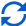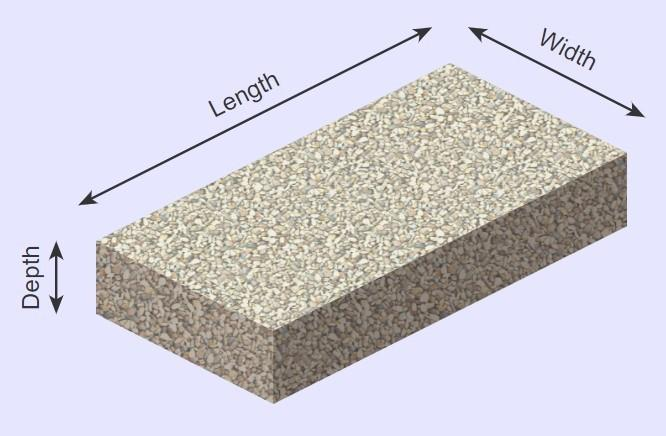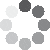Enter parameters LENGTH, WIDTH & DEPTH
Enter parameters
LENGTH, WIDTH & DEPTH
Length:
ft
• inches (in)
• feet (ft)
• yards (yd)
• centimeters (cm)
• meters (m)
Width:
ft
• inches (in)
• feet (ft)
• yards (yd)
• centimeters (cm)
• meters (m)
Depth:
in
• inches (in)
• feet (ft)
• yards (yd)
• centimeters (cm)
• meters (m)
Density:
105 - Gravel lb/ft³
• Gravel - 105 lb/ft³
• Concrete - 150 lb/ft³
• Limestone - 160 lb/ft³
• Sandstone - 145 lb/ft³
• Granite - 168 lb/ft³
• Marble - 160 lb/ft³
• Slate - 168 lb/ft³
• Basalt - 188 lb/ft³
• Crushed stone - 100 lb/ft³
• Sand - 100 lb/ft³
• Topsoil - 110 lb/ft³
• Custom
Price per unit of mass:
t
• pound (lb)
• US short ton (t)
• imperial-long ton (long t)
• kilogram (kg)
Price per unit of volume:
yd³
• cubic yards (yd³)
• cubic feet (ft³)
• cubic inches (in³)
• cubic centimeters (cm³)
• cubic meters ()Result
Area:
0
ft²
• square feet (ft²)
• square inches (in²)
• square yards (yd²)
• square miles (mi²)
• acres (ac)
• square centimeters (cm²)
• square meters ()
Volume:
0
yd³
• cubic yards (yd³)
• cubic feet (ft³)
• cubic inches (in³)
• cubic centimeters (cm³)
• cubic meters ()
Weight:
0
t
• pounds (lb)
• US short tons(t)
• imperial-long tons (long t)
• kilograms (kg)
Cost:
$Enter parameters AREA & DEPTH Area: ft² • square feet (ft²) • square inches (in²) • square yards (yd²) • square miles (mi²) • acres (ac) • square centimeters (cm²) • square meters () Depth: in • inches (in) • feet (ft) • yards (yd) • centimeters (cm) • meters (m) Density: 105 - Gravel lb/ft³ • Gravel - 105 lb/ft³ • Concrete - 150 lb/ft³ • Limestone - 160 lb/ft³ • Sandstone - 145 lb/ft³ • Granite - 168 lb/ft³ • Marble - 160 lb/ft³ • Slate - 168 lb/ft³ • Basalt - 188 lb/ft³ • Crushed stone - 100 lb/ft³ • Sand - 100 lb/ft³ • Topsoil - 110 lb/ft³ • Custom Price per unit of mass: t • pounds (lb) • US short tons (t) • imperial-long tons (long t) • kilograms (kg) Price per unit of volume: yd³ • cubic yards (yd³) • cubic feet (ft³) • cubic inches (in³) • cubic centimeters (cm³) • cubic meters ()Result Volume: 0 yd³ • cubic yards (yd³) • cubic feet (ft³) • cubic inches (in³) • cubic centimeters (cm³) • cubic meters () Weight: 0 t • pounds (lb) • US short tons (t) • imperial-long tons (long t) • kilograms (kg) Cost:$
Enter parameters
VOLUME
Volume:
yd³
• cubic yards (yd³)
• cubic feet (ft³)
• cubic inches (in³)
• cubic centimeters (cm³)
• cubic meters ()
Density:
105 - Gravel lb/ft³
• Gravel - 105 lb/ft³
• Concrete - 150 lb/ft³
• Limestone - 160 lb/ft³
• Sandstone - 145 lb/ft³
• Granite - 168 lb/ft³
• Marble - 160 lb/ft³
• Slate - 168 lb/ft³
• Basalt - 188 lb/ft³
• Crushed stone - 100 lb/ft³
• Sand - 100 lb/ft³
• Topsoil - 110 lb/ft³
• Custom
Price per unit of mass:
t
• pounds (lb)
• US short tons (t)
• imperial-long tons (long t)
• kilograms (kg)
Price per unit of volume:
yd³
• cubic yards (yd³)
• cubic feet (ft³)
• cubic inches (in³)
• cubic centimeters (cm³)
• cubic meters ()Result
Weight:
0
t
• pounds (lb)
• US short tons (t)
• imperial-long tons (long t)
• kilograms (kg)
Cost:
$(No Ratings Yet)Loading... Similar Calculators: Real-time graphics. Make the calculations and see the changes. Real-time graphics. Make the calculations and see the changes. Real-time graphics. Make the calculations and see the changes. Embed Similar Calculators:We think you will agree when we say that guessing how many pounds of crushed stone your landscaping construction will require is not easy. Estimating by eye is ineffective and can result in you wasting time and money purchasing unnecessary amounts of material. If that sounds familiar, then you need our aggregate calculator. In this article we will show you just how easy it is to use and understand. We will also detail the formulae the calculator uses and take you through some examples. 👍 Contents: ## Aggregate calculator formulaThe calculator first works out the area and volume of the space using the measurements you provide. In addition, it calculates the total weight if you know the density of the crushed material. It calculates these values using the formulae: $$Area = Length \times Width$$ $$Volume = Area \times Depth$$ $$Weight = Density\,of\,aggregate \times Volume$$ NB: Our calculator has many standard densities already programmed for you – for example, gravel (105 lb/ft³), concrete (150 lb/ft³), sand (100 lb/ft³), and many more. Click on the drop down menu to see all the options! But, there’s more! If you know the price per unit mass/volume of the crushed material, then the calculator can also work out the total cost of the aggregate using the formula: $$Cost = Price\,per\,unit\,mass \times Weight$$ or $$Cost = Price\,per\,unit\,volume \times Volume$$ Of course, you might not know the price per unit mass/volume. If so, then you can calculate this yourself using the formula: $$Price\,per\,unit\,mass = {Total\,cost\,of\,aggregate \over Weight\,of\,aggregate}$$ or $$Price\,per\,unit\,volume = {Total\,cost\,of\,aggregate \over Volume\,of\,aggregate}$$ Something not clear? 🤔 See below for three full examples detailing how the calculator gives you the volume, weight and cost. Otherwise, simply enter your measurements and correct units in the calculator to start your next outdoor construction project efficiently! ## Example calculations • Fill a space with concrete (150 lb/ft³) Let’s say we need to fill in an area of space measuring 5 feet long, 3 feet wide and 2 inches deep with concrete which costs$50 per US short ton.

I want to work out an estimate for the volume of concrete required, how much it will weigh and the total expected cost of the project.

I would enter these measurements into the calculator, which would perform the following operations:

$$Area = Length \times Width = 5\,ft \times 3\,ft = 15\,ft^2$$

$$Volume = Area \times Depth = 15\,ft^2 \times 2\,in = 2.5\,ft^3$$

$$Weight = Density\,of\,aggregate \times Volume = 150\,lb/ft^3 \times 2.5\,ft^3 = 375\,lb$$

$$Cost = Price\,per\,unit\,mass \times Weight = 50\,/t \times 375\,lb = 9.38$$

• Fill 12 square feet with custom aggregate

For the second example, let’s now say that I have an area of 12 ft² that I want to fill with an aggregate material.

The base is to a depth of 4 inches.

I will use a custom material of unknown density which weighs 200 lb per four cubic feet and costs $60 per US short ton. First, in order to calculate the material’s density, I would do the following calculation: $$Density = {Mass \over Volume} = {200\,lb \over 4\,ft^3} = 50\,lb/ft^3$$ I would then enter the measurements into the online estimator to work out the volume, weight and total cost: $$Volume = Area \times Depth = 12\,ft^2 \times 4\,in = 4\,ft^3$$ $$Weight = Density\,of\,aggregate \times Volume = 50\,lb/ft^3 \times 4\,ft^3 = 0,1\,t$$ $$Cost = Price\,per\,unit\,mass \times Weight = 60\,/t \times 0,1\,t = 6$$ • Fill 5 cubic feet calculating price per unit volume In our final example, let’s imagine I need to fill 5 ft³ with gravel (105 lb/ft³). I don’t know the price per unit volume but I can purchase 20 cubic yards of gravel for$250.

I can therefore calculate the price per unit volume of the stones using the method:

$$Price\,per\,unit\,volume = {Total\,cost\,of\,aggregate \over Volume\,of\,aggregate} = {250 \over 20\,yd^3} = 12.5 \,/yd^3$$

I therefore enter the measurements into the calculator which does the following operations to work out the weight and cost of the crushed stones:

$$Weight = Density\,of\,aggregate \times Volume = 105\,lb/ft^3 \times 5\,ft^3 = 525\,lb$$

$$Cost = Price\,per\,unit\,volume \times Volume = 12.5 \,/yd^3 \times 525\,lb = 2.32$$

## What if my measurements are in different units?

Finally, at this point it’s likely you are wondering what to do if you are working with different units.

You may have noticed that in the above examples we change between tons and pounds, feet and yards and so on.

Our online calculator is able to do the conversions for you, saving you time and effort! ⏱️

Simply select the correct unit in the drop down area and the calculator will do the following conversions for you:

$$1\,foot = 12\,inches = 0.33\,yards = 30.48\,centimeters = 0.3048\,meters$$

$$1\,US\,short\,ton = 2000\,pounds = 0.893\,imperial\,long\,ton = 907\,kg$$

It’s that easy! 😃

Embed this calculator on your site!Add live graphics
Copied to clipboard! Preview